Lei, J., Liu, Z., Ren, D., Wang, S., Lei, D., Luo, J., and Lei, M. (2023). “Discrete elemental parameter calibration of stacking behavior of sugarcane tail leaf sieved material,” BioResources 18(3), 4834-4849.

#### Abstract

To improve the accuracy of discrete element simulation parameters of sugarcane tail-leaf (STL) feed during dust removal and crushing, this study used a combination of physical tests and EDEM software simulations to calibrate the discrete element simulation parameters of crumbs and dust in the feed. Taking the experimental physical stacking angle (SA) as the response value, the second-order regression models of SA and significant factors were established by Plackett-Burman test, steepest climb test, and Box-Behnken test. Variance analysis and interaction effect analysis were conducted. Taking the accumulation angle of 41.27° obtained by physical experiments as the target value, the significant parameters were optimized. The optimal combination of the following parameters was obtained: tail stem-dust static friction coefficient (SFC) of 0.46, tail leaf-dust coefficient of sliding friction (COSF) of 0.205, JKR surface energy of 0.26, and dust-steel collision recovery coefficient (CRC) of 0.338. Through software simulation verification, the average value was 40.81°, and the relative error of the SA with the physical experiment was 1.13%. The results showed that the calibrated parameters are real and reliable, which can provide a theoretical reference for the design optimization of the straw crushing device, feed processing device, and other related components.

Discrete Elemental Parameter Calibration of Stacking Behavior of Sugarcane Tail Leaf Sieved Material

Junle Lei,* Zhaochong Liu, Danni Ren, Shuaiwei Wang, Dingyuan Lei, Jiawei Luo, and Ming Lei

To improve the accuracy of discrete element simulation parameters of sugarcane tail-leaf (STL) feed during dust removal and crushing, this study used a combination of physical tests and EDEM software simulations to calibrate the discrete element simulation parameters of crumbs and dust in the feed. Taking the experimental physical stacking angle (SA) as the response value, the second-order regression models of SA and significant factors were established by Plackett-Burman test, steepest climb test, and Box-Behnken test. Variance analysis and interaction effect analysis were conducted. Taking the accumulation angle of 41.27° obtained by physical experiments as the target value, the significant parameters were optimized. The optimal combination of the following parameters was obtained: tail stem-dust static friction coefficient (SFC) of 0.46, tail leaf-dust coefficient of sliding friction (COSF) of 0.205, JKR surface energy of 0.26, and dust-steel collision recovery coefficient (CRC) of 0.338. Through software simulation verification, the average value was 40.81°, and the relative error of the SA with the physical experiment was 1.13%. The results showed that the calibrated parameters are real and reliable, which can provide a theoretical reference for the design optimization of the straw crushing device, feed processing device, and other related components.

DOI: 10.15376/biores.18.3.4834-4849

Keywords: Cane tail leaf; Dust extraction; Discrete element simulation; Stacking angle

Contact information: College of Mechanical and Control Engineering, Guilin University of Technology, Guilin, 541004, P. R. China; *Corresponding author: 40911409@qq.com

INTRODUCTION

Sugarcane is a cash crop distributed in tropical and subtropical regions, and it is mainly cultivated in the southern and southwestern regions of China (Huang 2020). The Guangxi region of China has the largest planting area, with an annual planting area of 16 about million mu, making up 63% of the country’s plantings. The top 2 to 3 tender nodes of sugarcane culms and their entire leaves are called the STL, and they have an annual yield of 15 million tons in Guangxi alone (Zhou et al. 2021). STL is a medium quality roughage (Wang et al. 2018), with more comprehensive nutrition and low cost, making it an excellent silage resource.

Various factors, such as technology and equipment, lead to the on-site burning of a large amount of STL produced after the annual harvest, with less than 10% utilization (Liang 2008; Zhou et al. 2019). Rational use of STL would solve the problem of lack of feed for livestock in winter, save a lot of food, reduce the cost of breeding, and reduce air pollution caused by burning straw. Therefore, it is economically and socially important to improve the mechanized recovery of cane leaves. The current straw feed grinder collects straw from the ground and crushes it directly into feedable feed. During the picking process, the device includes soil and other impurities in the crushed feed, which reduces the cleanliness of the straw feed, making it less palatable. This has a direct impact on the later feed fermentation and mold, etc. Therefore, it is significant to study how to dust and dust reduction treatment of straw raw materials before harvest to improve the cleanliness and quality of straw feed.

With the development of agricultural machinery, current straw harvester equipment with dust removal devices is also increasing year by year. This includes work by Ma et al. (2020) for the design of corn straw crushing and dust removal devices. Zhang et al. (2020) proposed the use of a combination of electrostatic and spray dust removal to reduce the problem of dust at wheat harvesting sites. Li (2020) worked on the development of straw harvester screw conveyor dust removal device. Although the usage of harvester equipment that incorporates dust removal function is gradually increasing, dust removal and straw material separation mechanism research is still relatively rare. Therefore, the study of the separation mechanism of straw materials and the movement law of dust during the crushing process is of decisive significance for the design and improvement of optimized dust removal devices to improve dust removal efficiency.

The study of modern devices with dust removal requires the selection and verification of the relevant material contact parameters before design. Accurate acquisition of the parameters is a prerequisite for the application of the discrete element method to study the material properties. In recent years, with the advance of science and technology, the finite element method has been increasingly applied to the research field of agricultural science (Bai et al. 2013). Foreign scholars have applied the finite element method to study the mechanical properties of agricultural materials and achieved many scientific results (Lu et al. 2006; Onder et al. 2008; Liu et al. 2020; Huan et al. 2022).

Domestic researchers have also studied the application of the finite element method to a variety of crop materials: for example, the calibration of mung bean seed-to-glass simulation parameters by Zhang et al. (2022); Liu et al. (2018) for the calibration of the simulation parameters between the miniature potato and the contact steel plate; Ma et al. (2020) calibrated the contact parameters between alfalfa straw and contact steel plates; Wen et al. (2020) calibrated and validated the contact parameters between the sugarcane material and the steel plate.

In summary, there have been more research results on the calibration of discrete meta-simulation data with straw material or plant seed as the research object, but the research on the physical parameters and simulation parameters between material debris and dust impurities is relatively rare. Therefore, in this paper, a mixture of straw debris and dust in STL feed was studied, and the discrete element simulation parameters were calibrated by being combined with physical tests and software data simulation such as EDEM. The mathematical models of SA and related parameters were set by Plackett-Burman test screening. Steepest climb test and Box-Behnken test analysis, and the related parameters were optimized.

The results showed that it is feasible to apply the above combined experiments to calibrate the discrete element simulation parameters, with a view to providing a theoretical reference for the mechanistic study and optimization of the mechanical device for the separation of dust impurities and straw material movements.

EXPERIMENTAL

Materials

The experimental material was selected from the experimental field of Guilin Branch of Guangxi Academy of Agricultural Sciences, and sifted material of green storage feed after pulverizing STL was taken as the research object. Twenty feed samples were randomly selected, each of which was 200 g. According to GB/T5917.1 (2008), steel standard screens with mesh diameters of 3 mm and 7 mm were selected to manually screen straw feed, and the sifted material contained straw debris, soil, and other impurities. Due to the relatively small proportion of other impurities, this experiment was ignored. The average diameter and length of crushed material measured by electronic Vernier caliper was 2 mm, and the average length was 5.5 mm. The diameter of the dust particles was 0.5 mm. The sifted debris was collected and weighed. The measured mass was 487.2 g, representing 12.18% of the total mass. Straw crumbs were screened again. The material crumbs were separated from the dust, and the dust mass was 43 g, representing 8.83% of the material. The mixture is dried using a drying oven (Shanghai Jinghong Experimental Equipment Co., Ltd.). The drying oven was dried by vertical convection. Weighing was performed with an electronic scale, and the moisture content of the mixture before sieving was measured to be about 62%, and the moisture content of the mixture after sieving was about 50%. The drying oven equipment is shown in Fig. 1.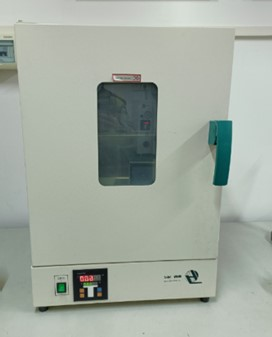Fig. 1. Drying Oven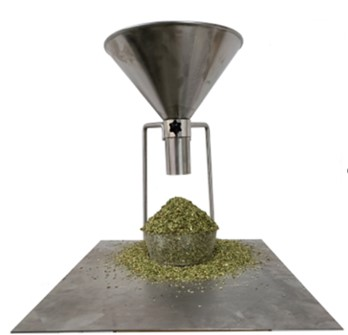Fig. 2. Accumulation angle measuring device

Stacking Angle Measurement Experiment

The material stacking angle was measured using the funnel method. A self-made steel funnel was used, with an overall height of 250 mm, a diameter of 240 mm at the top of the funnel, and a diameter of 55mm at the bottom of the outlet. The funnel and the funnel holder were placed on a horizontal table, with a round transparent container of 110 mm in diameter and 50 mm in height directly below the funnel mouth, and the funnel outlet was 160 mm high from the table. Screened crushed materials were poured into the funnel at a uniform speed. SA experimental measurement device was as shown in Fig. 2.

After the material accumulation angle had been basically set, the camera was used to photograph the front facing image of the material pile horizontally. Then the images were imported into MATLAB software (MathWorks Ltd., v.2018a, Massachusetts, USA) and the images are analyzed and processed using image digitizing tools. The above experiment was repeated 10 times, and the physical SA of the material was obtained at 41.27°. MATLAB software processing was as shown in Fig. 3.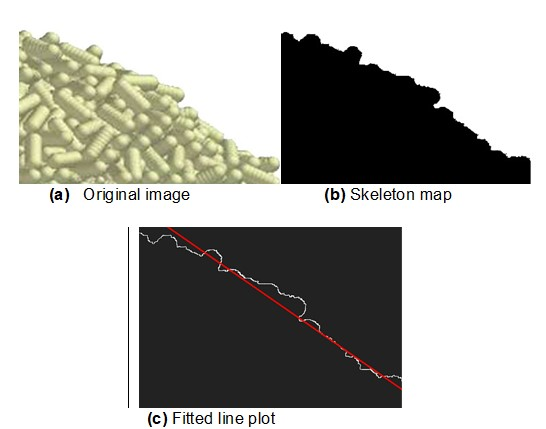Fig. 3. Linear fit by MATLAB software

Static Friction Coefficient (SFC) Test

This experiment used the ramp method. The SFC of tail stem-dust and tail leaf-dust were determined using the ramp meter, as shown in Eqs. 1 and 2,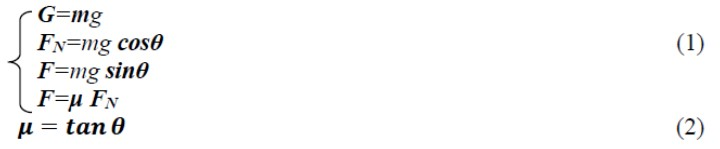In the above equation, G is the vertical downward force of gravity (N); FN is the normal support force of the vertical inclined plane (N); F is the frictional force in the direction opposite to the direction of motion (N); μ is the SFC; m is the mass of the object (kg); G is the acceleration of gravity (m/s2); and θ is the angle of inclination (°). According to the orthogonal decomposition of the force, the mathematical relationship between the coefficient of friction and the angle of inclination can be obtained from the formula (2). According to Lei et al. (2022), the STL were divided into two parts: tail stems and tail leaves, and 10 of each part were selected as samples. Shear lengths of the caudal stem and caudal leaf were 40 mm and 50 mm, respectively. The container and the inclinometer were fixed together and placed on a horizontal table. The arid soil taken from the experimental field was filled with a transparent container and the surface was leveled. Then the experimental sample was measured and placed on the soil. The inclinometer was slowly rotated. Rotation was stopped when the material began to appear to slide evenly, and the angle of inclination was recorded at this time. Using this method, the experiment was repeated 20 times, and the average value was calculated. The SFC of tail stem and dust and tail leaf and dust was found to be 0.3~0.7 and 0.2~0.7, respectively.

Coefficient of Sliding Friction (COSF) Test

Using a similar method of measuring the SFC, the COSF of the dust-tail stem and the dust-tail leaf was measured using an inclinometer. The material was placed on the soil surface, and the inclinometer was turned slowly. Turning was stopped when the material started to roll, and the corresponding angle was recorded. The experiment was repeated 20 times, and the average value of COSF for tail stem and dust or tail leaf and dust was 0.1 to 0.5 or 0.1 to 0.6, respectively. The measured value of the angle varied widely during the experiment. To ensure the accuracy of the numerical parameters, the range of data variation obtained from the test was used as the basis for selecting the parameters of the discrete element simulation test. Finally, the best value of the parameters was determined by numerical simulation.

DISCRETE ELEMENT MODEL BUILDING

Selection of Contact Model

The sieved STL feed is silage, and there is a certain moisture content, so adhesion phenomenon will exist between the material particles. The surface energy in the JKR model can better reflect the bonding force between simulated particles, so the Hertz-Mindlin with JKR Cohesion contact model was chosen in this paper (Tian et al. 2021; Wang et al. 2021). The JKR planar bonding model is shown in Fig. 4.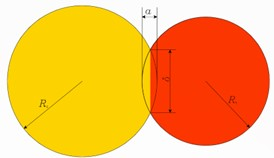Fig. 4 Schematic diagram of JKR bonding model

It is created on the basis of Hertz theory incorporating a particle contact model with cohesive gravity. Considering the influence of the adhesion force between wet particles on the particle motion pattern, it was applied to simulate the materials where adhesion and aggregation occur between particles with electrostatic and moisture factors, such as crops and soil. To reflect the existence of adhesion attraction between particles, the model applies the JKR normal elastic force FJKR as follows: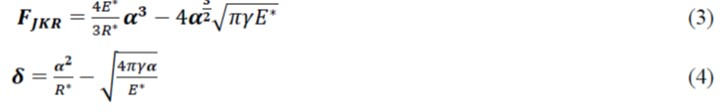where FJKR is the JKR normal elastic force (N); E* is the equivalent modulus of elasticity (Pa); α is the tangential overlapping of the particles in contact with each other (m); R* is the equivalent contact radius (m); δ is the amount of overlapping normal to the two contacting particles (m); and is the surface energy (N/m). The equivalent modulus of elasticity and equivalent contact radius is defined as follows,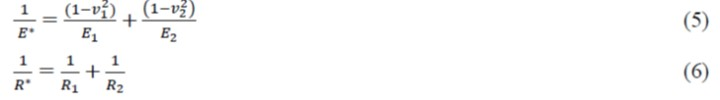where E1, ν1, R1, E2, ν2, and R2 are the modulus of elasticity, Poisson’s ratio (PR), and the radius of the two granular materials in contact with each other, respectively. When , the JKR normal force and the Hertz-Mindlin normal force FHertz are the same, i.e., the force becomes Hertz-Mindlin normal force, as shown in Eq. 7.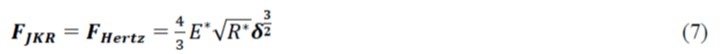Even if the particles are not in direct contact with each other, this model provides attractive adhesion, and the maximum distance at which cohesion exists between particles is calculated as follows,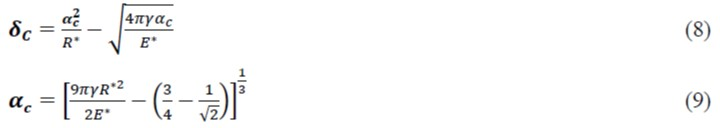In the above equation δc is the tangential maximum gap when there is non-zero cohesion between particles (m); is the normal maximum gap when there is non-zero cohesion between particles (m). When there is no actual contact between particles and the gap is less than , the cohesion reaches its maximum value: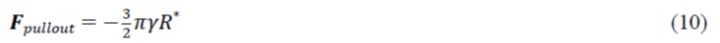When the JKR model is chosen to simulate particles with relatively high water content, the force required to separate between the 2 particles depends on the surface energy and the contact angle, calculated as follows,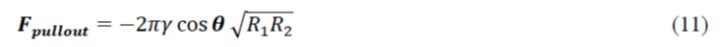where θ is the contact angle of the two particles. The frictional force of the Hertz-Mindlin with JKR Cohesion contact model is taken from the positive repulsive component of the JKR normal force. Therefore, a larger cohesion component of the contact force in this model results in a larger friction force.

The water content of green storage crushed sugarcane tail leaf feed itself is high, and there is a certain adhesion phenomenon between the mixed crushed particles affected by water molecules. The surface energy in the JKR model can better reflect the bonding force between simulated particles, so the Hertz-Mindlin with JKR contact model was chosen for the experiments in this paper, and the subsequent parameter calibration was carried out accordingly.

Discrete Element Modeling

The material for this experiment contains sugarcane tail stem, tail leaf, and dust. Because two types of straw shreds were similar in shape after sieving with minimal differences, the modeling was represented by the same length of cylindrical particles in the EDEM simulation software (DEM Solutions Ltd., v.2018, Edinburgh, UK). The particle model of both straw shreds was set as a cylinder of 5.5 mm in length and 2 mm in diameter, and the dust model was set as a sphere of 0.5 mm in diameter, as shown in Fig. 5.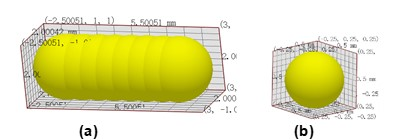Fig. 5. Numerical simulation model of tail stem, tail leaf (a) and dust (b)

The 3D funnel model and container model were imported and modeled by Solidwors software (Dassault Systemes S.A, v.2018, Concord, MA, USA). Proportionally the diameter of the upper part of the funnel was set to 144 mm, the diameter of the lower part was set to 16.5 mm, and the overall height was set to 150 mm. A circular container with a diameter of 66 mm and a height of 28 mm was set at 96 mm directly below the funnel outlet. The idea was to create a virtual particle factory of the same size as the funnel and set the particle factory to dynamic mode. Based on the ratio of straw fragments to dust, the mass of both the tail stems and tail leaves produced was set at 23.5 g, and the mass of dust produced was 4.15 g. The particles were set to be generated in a random manner. Production rates of 94 g/s and 36.5 g/s were selected for two types of particles, shredded and dusty, respectively, with a total time of 1 s. The particle drop speed was set to -1 m/s, the time step was set to 20%, and the default grid size of 2.5R was selected. The SA simulation model is presented in Fig. 6. After the pellet pile was stabilized, the angle measurement was performed using the software post-processing tool, and the pile angle measurement is illustrated in Fig. 7.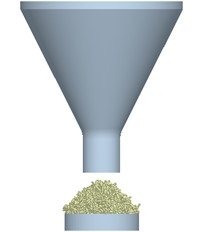Fig. 6. SA simulation experiment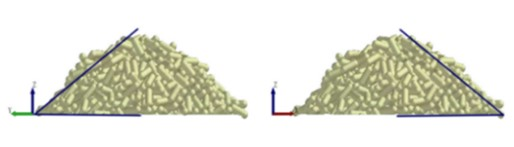Fig. 7. SA measurement

Table 1. Plackett-Burman Test Parameters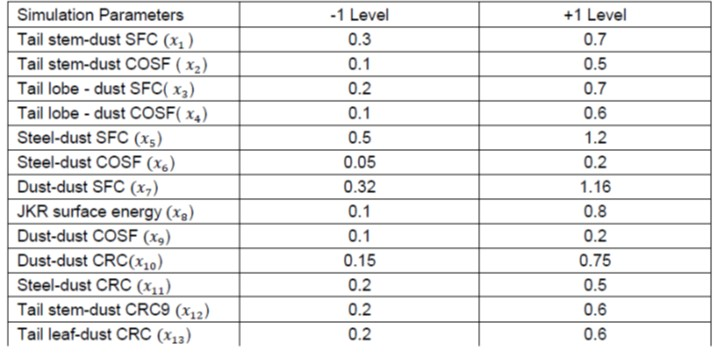Plackett-Burman Test

Experimental design for parameter calibration

The sieved material of this experiment contains dust, tail stem and tail leaf, so the contact parameters between the materials needed to be determined. Part of the data was obtained in the preceding material experiment. Further data were obtained by referring to the GEMM database and consulting related literature (Liu et al. 2016; Zhang et al. 2017; Zheng et al. 2017; Wang et al. 2021). Table 1 shows the parameter value ranges that were obtained.

Screening of Significance Factors

The Plackett-Burman test design was performed using the design-Expert12 design software against the parameters obtained in the test and the parameters selected from the literature review. Multi-factor significance analysis was performed using the SA as the response value to screen out the factors that had a significant effect on the response value. For each physical parameter, 1 high and 1 low level were selected, denoted by the codes (+1) and (-1), with the highest value of each parameter as the (+1) level and the lowest value as the (-1) level. One focal point was selected for this experiment and 21 trials were conducted. The symbols 𝑥1 to 𝑥13 denote each corresponding factor, and the design scheme and results are shown in Table 2.

The data from the Plackett-Burman test were subjected to ANOVA and ranked for significance of effect, as shown in Table 3. Tail stem-dust SFC (𝑥1), tail leaf-dust COSF (𝑥4), and steel-dust CRC (𝑥11) achieved P-values below 0.01, indicating a highly significant effect on the pile-up angle. The JKR surface energy coefficient (𝑥8) achieved P<0.05, indicating a significant effect on the SA. For other factors, it was found that P>0.05, indicating that the effects on SA were not significant. To simplify the experimental steps, only these four meaningful factors were analyzed in the subsequent Box-Behnken test, and the average value of the other non-significant factors was taken as the parameter value of the subsequent experiment.

Table 2. Plackett-Burman Test Protocol and Results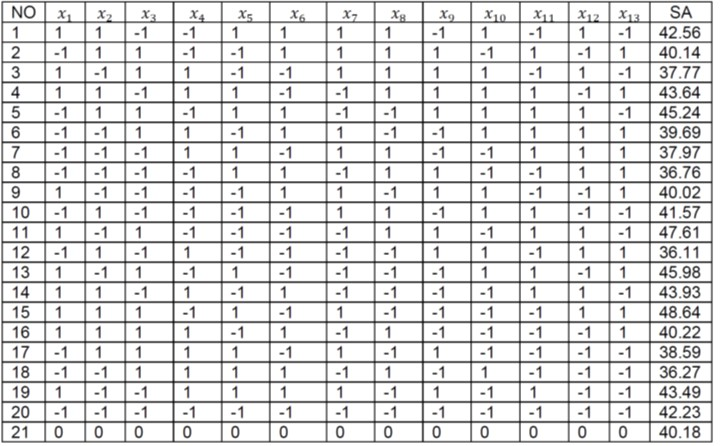Table 3. Plackett-Burman Test Variance and Significance Analysis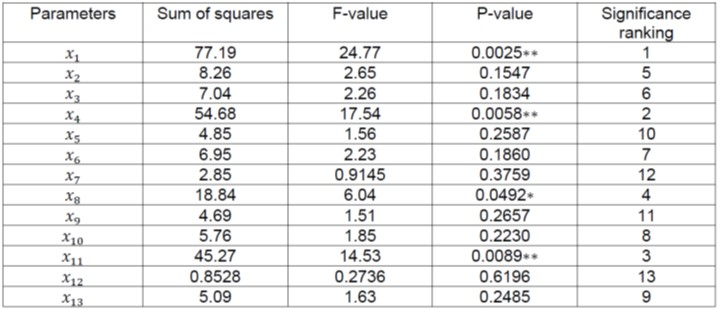Note: means that the factor is highly significant (P<0.01); means that the factor is significant (0.01<P<0.05); P>0.01 indicates that the factor is not significant, as follows.

Steepest Climb Test

The steepest climbing experiment was performed for four significant factors based on the results of the Plackett-Burman test. Using the relative error between the experimental physical stacking angle and the simulated experimental stacking angle as the response index, the optimal range of values for each factor can be accurately determined, which will greatly reduce the error. The steepest climbing experiments and results is shown in Table 4. The experimental results showed that the relative error gradually decreased as the parameter values increased. By the third group of experiments the relative error value was the smallest, so the best parameter was near the third group of experimental data. The third set of experimental parameters was taken as intermediate level values, and the second and fourth sets of data were taken at low and high levels for subsequent Box-Behnken tests, respectively.

Table 4. Steepest Climbing Test and Result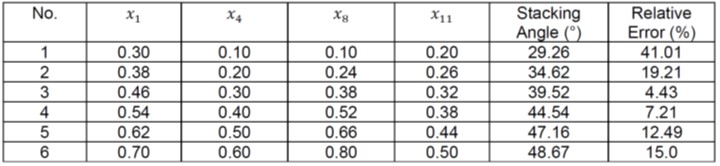Box-Behnken Test Design and Analysis

To find the optimal combination of parameters that significantly affect the factors in the simulation experiment, the Box-Behnken experimental design was carried out by taking the second, third, and fourth groups of experimental parameters as the corresponding low, medium, and high levels according to the steepest climbing experimental data in turn.

Table 5. Box-Behnken Test Design and Results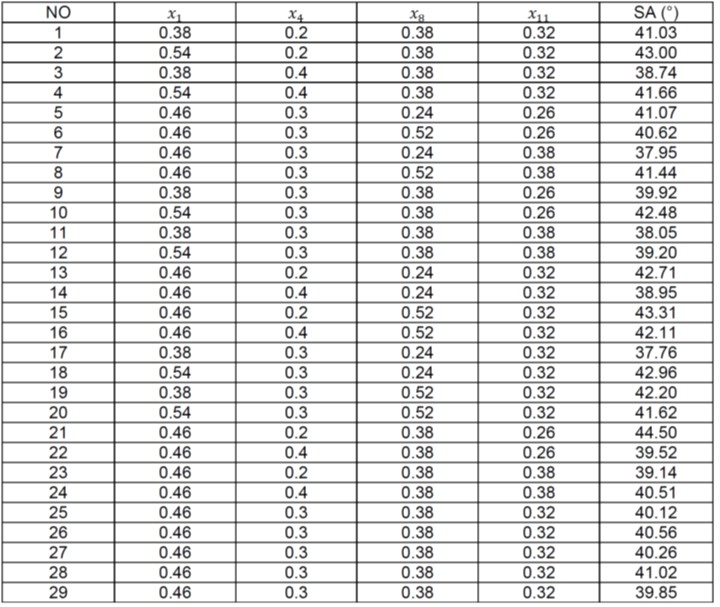A four-factor, three-level experimental design was conducted with the SA as the response value, and five center points were set by default. A total of 29 sets of experiments were conducted. The experimental design scheme and results are shown in Table 5.

Multivariate fitting and ANOVA were performed on the experimental results using Design-Expert12 software to establish a second-order regression model between the SA and the functions of the four independent variables, and the quadratic regression equation was: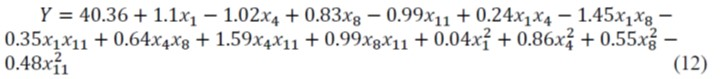The regression model of the experimental design was subjected to ANOVA, and the results of the analysis are shown in Table 6. The regression model achieved P<0.0001, indicating that the effect of this simulation model was extremely significant and can accurately reflect the relationship between the independent and dependent variables. The coefficient of determination R2=0.9652 and the corrected coefficient of determination R2adj = 0.9304, both were close to 1, indicating that the simulated experiment fits well with the actual experiment; P=0.5255>0.05 for the failure to fit term, indicating that there was no effect of other extraneous factors. The coefficient of variation of C.V. =1.12%, indicating the high reliability of the experiment. The test accuracy AP=20.5475 indicates that the model is highly accurate and can accurately reflect the target SA. P-values for the single factors 𝑥1, 𝑥4, 𝑥8, 𝑥11 and the interaction terms 𝑥1 𝑥8, 𝑥4 𝑥11, 𝑥8 𝑥11 and the squared terms, were less than 0.01, indicating that these parameter values have a highly significant effect on the response value of SA.

Table 6. Box-Behnken Experimental Design and Regression Model Analysis of Variance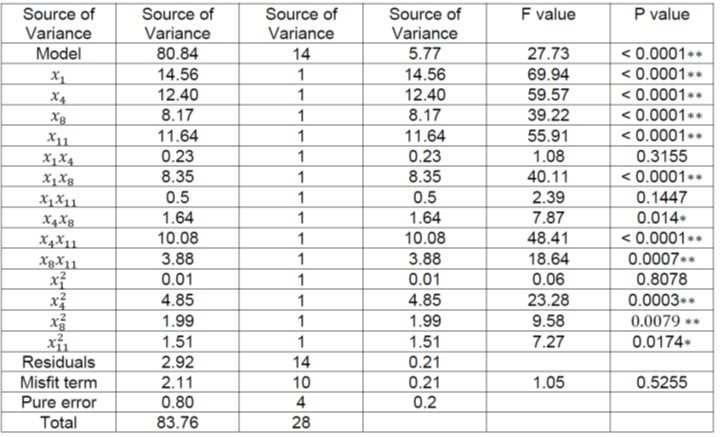The P-value for the interaction term was less than 0.05, indicating that this interaction term parameter had a significant effect on the response value of SA. The p-values of the interaction term 𝑥1 𝑥4, 𝑥1 𝑥11 and the squared term were all greater than 0.05, indicating that the effect of this factor on the SA was not significant. Removing the non-significant factors, the optimized quadratic regression equation was determined (Eq. 13).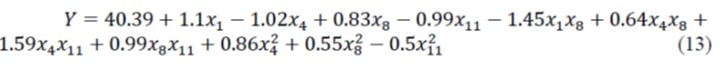Regression Model Interaction Effect Analysis

Based on the data in Table 6, it can be seen that the interaction terms 𝑥1𝑥8, 𝑥4𝑥8, 𝑥4𝑥11, 𝑥8𝑥11 all have a significant effect on the material of SA. To further analyze the effect of each factor of the interaction term on the SA, the 3D response surface of the interaction term was analyzed using Design-Expert software, and the effect of the interaction term factors on the SA is shown in Fig. 8.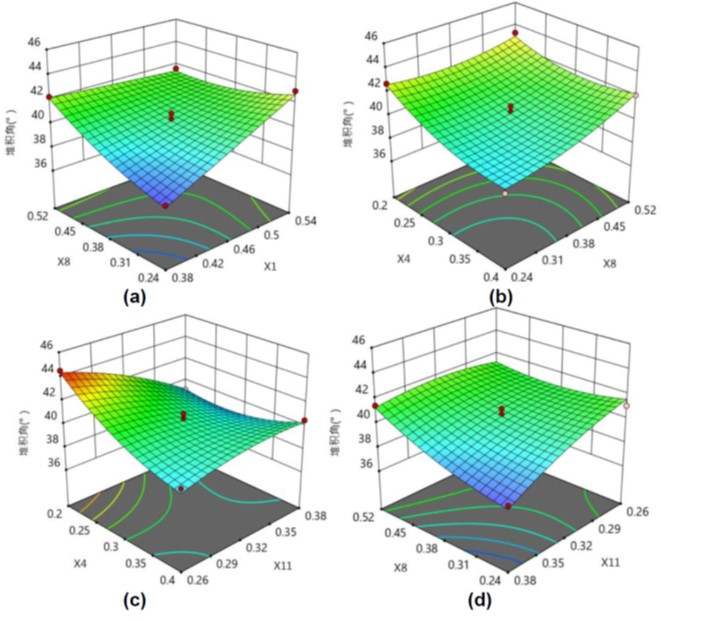Fig. 8. The effect of interaction on SA

In Fig. 8(a), the slopes of the response surface curve of 𝑥1 and 𝑥8 are similar, indicating that the two significant factors affect the SA with basically the same degree of significance. In Fig. 8(b), the contour line density is somewhat greater in the 𝑥4 directions and the response surface curve are slightly steeper, indicating a more significant effect on the SA than 𝑥8. In Fig. 8(c), the contour lines are significantly denser in the 𝑥4 direction and the slope is obvious, indicating that 𝑥4 has a more significant effect on the SA than 𝑥11. In Fig. 8(d), 𝑥8 its contour density is biased higher than that along the direction of 𝑥11, indicating that 𝑥8 has a more significant effect on the SA than 𝑥11.

Verification of Optimal Parameter Combinations

The optimization function of Design-Expert software was used to take the average value of a physical test 41.27° as the target value, and the first few groups recommended in the system were selected as the verification objects for simulation. The closest set of data to the target value was selected as the optimal value, i.e., 𝑥1 is 0.46, is 0.205, 𝑥8 is 0.26, and 𝑥11 is 0.338. To verify the accuracy of the selected parameters, three more numerical simulations of the SA were performed for this combination of parameters, and the average value of the simulation results was 40.81°, with a relative error of 1.13% with the actual physical SA. The physical and simulation experiments are compared in Fig. 9.